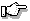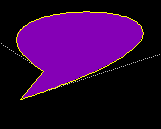# PgDrawBezier(), PgDrawBeziermx()

Draw a stroked and/or filled bézier

## Synopsis:

```int  PgDrawBezier( PhPoint_t const *ptr,
int num,
PhPoint_t const *pos,
int flags );

int  PgDrawBeziermx( PhPoint_t const *ptr,
int num,
PhPoint_t const *pos,
int flags );```

## Description:

These functions build a command in the draw buffer to draw a multisegment Bézier curve from an array of points.

Each Bézier curve is defined by 4 points. The last point of a curve becomes the first point of the next curve. The first and fourth points are anchor points; the line passes through these. The second and third points are control points; the curve is "pulled" toward these points.

The flags argument must be one of the following:

• Pg_DRAW_STROKE - draw a stroked curve.
• Pg_DRAW_FILL - draw a filled curve.
• Pg_DRAW_FILL_STROKE - draw a filled curve, then stroke it.

You can OR flags with any combination of the following:

• Pg_CLOSED - connect the last point to the first.
• Pg_RELATIVE - use relative coordinates to draw the curve. Each point is relative to the previous point.

For absolute coordinates, pos is added to each point pointed to by ptr. For relative coordinates, the first coordinate is the sum of pos and the first point of the array; any subsequent coordinate is the sum of the previous point and the next point of the array.If you call the "mx" form of this function, the data isn't physically copied into the draw buffer. Instead, a pointer to the array is stored until the draw buffer is flushed. Make sure you call PgFlush() before you modify the point array.

## Returns:

0
Success.
-1
The draw buffer is too small to hold the current draw state, the draw command, and the data. Increase the size of the draw buffer or decrease the number of points.

## Examples:

The following example:

```DrawFillStrokeBezier() {
PhPoint_t   o = { 0, 0 };
PhPoint_t   p[] = {43, 71, -92, -18, 344, -6, 20, 99};

PgSetStrokeDash( "\1", 1, 0x10000 );
PgSetStrokeColor( Pg_GRAY );
PgDrawPolygon( &p, 4, &o,
Pg_DRAW_STROKE | Pg_CLOSED );
PgSetStrokeDash( NULL, 0, 0 );
PgSetStrokeColor( Pg_YELLOW );
PgSetFillColor( Pg_PURPLE );
PgDrawBezier( &p, 4, &o,
Pg_DRAW_FILL_STROKE | Pg_CLOSED );
}```

will draw:The dotted lines show where the control points are relative to the anchor points.

## Classification:

Photon

Safety:
Interrupt handler No
Signal handler No
Thread No

## See also:

To draw stroked Bézier curves, see also:

To draw filled Bézier curves, see also: